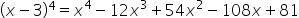Maths-
General
Easy

Question

# Use binomial theorem to expand (x - 3)4Hint:

## The correct answer is: (x - 3)4 = x4 - 12x3 + 54x2 - 108x + 81

### Step 1 of 2:Here, the value of n=4. So, the combination permutation we would use is 4Cr .We would have 4+1=5 terms in the expansion of the expression (x - 3)4Step 2 of 2:Substitute the values of (x - 3)4 in the binomial expansion:Thus, the expansion is:For the expansion of an expression (x + y) , we would have n+1 terms. This is something you need to keep in mind.#### With Turito Foundation.#### Get an Expert Advice From Turito.